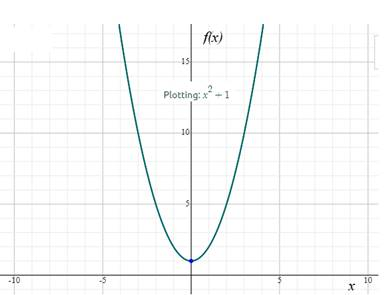# Find the domain of the function f ( x ) = x 2 + 1### Precalculus: Mathematics for Calcu...

6th Edition
Stewart + 5 others
Publisher: Cengage Learning
ISBN: 9780840068071### Precalculus: Mathematics for Calcu...

6th Edition
Stewart + 5 others
Publisher: Cengage Learning
ISBN: 9780840068071

#### Solutions

Chapter 2.1, Problem 44E
To determine

## Find the domain of the function f(x)=x2+1

Expert Solution

The domain of the function f(x)=x2+1 is (,)

### Explanation of Solution

Given information:Consider the function f(x)=x2+1

Calculation:

Recall the domain of a function is the set of input or argument values for which the function is real and defined.

Assume a function from real values to real values as shown in the figure below:From the above graph, given function have no undefined points.

Thus, the domain of the function f(x)=x2+1 is (,)

### Have a homework question?

Subscribe to bartleby learn! Ask subject matter experts 30 homework questions each month. Plus, you’ll have access to millions of step-by-step textbook answers!